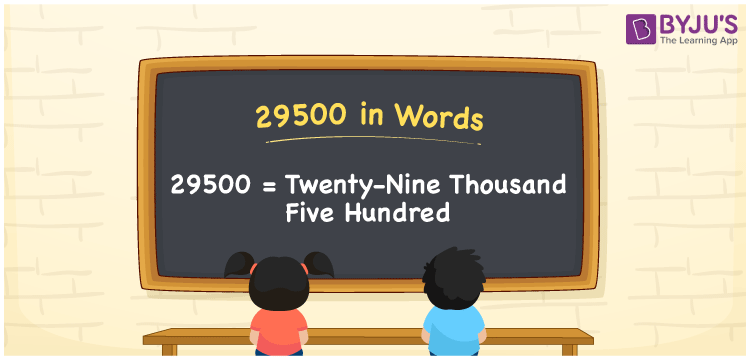# 29500 in Words

29500 in words is Twenty-nine thousand five hundred. For example, if you earned Rs. 29500 in a month, you can write, “I have earned Rs. Twenty-nine thousand five hundred in a month”. In general, the number name of any number can be written using the ones, tens, hundreds, and thousands place of a number. Thus, the place value chart is useful for writing the number 29500 in words.

 29500 in words Twenty-nine thousand five hundred twenty-nine thousand five hundred in Numbers 29500

## 29500 in English Words## How to Write 29500 in Words?

We can convert 29500 to words using a place value chart. This can be done as follows. The number 29500 has 5 digits, so let’s make a chart that shows the place value up to 5 digits.

 Ten thousands Thousands Hundreds Tens Ones 2 9 5 0 0

Thus, we can write the expanded form as:

2 × Ten thousand + 9 × Thousand + 5 × Hundred + 0 × Ten + 0 × One

= 2 × 10000 + 9 × 1000 + 5 × 100 + 0 × 10 + 0 × 1

= 29500

= Twenty-nine thousand five hundred

29500 is the natural number that is succeeded by 29499 and preceded by 29501.

29500 in words – Twenty-nine thousand five hundred

Is 29500 an odd number? – No.

Is 29500 an even number? – Yes

Is 29500 a perfect square number? – No

Is 29500 a perfect cube number? – No

Is 29500 a prime number? – No

Is 29500 a composite number? – Yes

## Frequently Asked Questions on 29500 in Words

Q1

### How to write 29500 in words?

29500 in English is written as “Twenty-nine thousand five hundred”.
Q2

### Is the number 29500 an odd number?

No, the number 29500 is not an odd number.
Q3

### Is 29500 a composite number?

Yes, 29500 is a composite number.